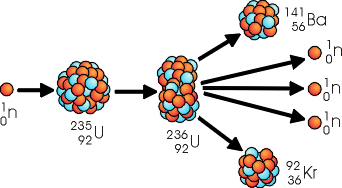# What is the definition of nuclear energy?

Mar 18, 2016

It's the energy produced by nuclear reactions.

#### Explanation:

Examples of famous nuclear reactions

${\quad}_{0}^{1} \text{n" + _92^235 "U" -> _56^141 "Ba" + _36^92 "Kr" + 3 _0^1 "n}$${\quad}_{1}^{2} \text{H" + _1^3 "H" -> _2^4 "He" + _0^1 "n}$Alpha decay

${\quad}_{92}^{238} \text{U" -> _2^4 "He" + _90^234 "Th}$${\quad}_{6}^{14} \text{C" -> _7^14 "N" + "electron} {+}_{0}^{0} \overline{v}$We use fusion reactions to generate energy currently. In the future, we may be using fission reactions to generate energy.